##### 4.1.3.2 So­lu­tion harmc-b

Ques­tion:

Ver­ify that there are no sets of quan­tum num­bers miss­ing in the spec­trum fig­ure 4.1; the listed ones are the only ones that pro­duce those en­ergy lev­els.

An­swer:

The generic ex­pres­sion for the en­ergy isor defin­ing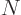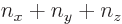,Now for the bot­tom level,0, and since the three quan­tum num­bers,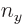, and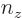can­not be neg­a­tive, the only way thatcan be zero is if all three num­bers are zero. If any one of,, orwould be pos­i­tive, then so would be. So there is only one state0.

For the sec­ond en­ergy level,1. To get a nonzero sum, you must have one of,, andto be nonzero, but not more than 1, orwould be more than 1 too. Also, if one of,, andis 1, then the other two must be 0 or their sumwould still be greater than 1. That means that pre­cisely one of,, andmust be 1 and the other two 0. There are three pos­si­bil­i­ties for the one that is 1,,, or, re­sult­ing in the three dif­fer­ent sets of quan­tum num­bers shown in the spec­trum fig­ure 4.1.

For the third en­ergy level,2, the max­i­mum value value any one of,, andcould pos­si­bly have is 2, but then the other two must be zero. That leads to the first three sets of quan­tum num­bers shown in the spec­trum. If the max­i­mum value among,, andis not 2 but 1, then a sec­ond one must also be 1, or they would not add up to 2. So in this case you have two of them 1 and the third 0. There are three pos­si­bil­i­ties for the one that is 0, pro­duc­ing the last three sets of quan­tum num­bers shown in the spec­trum 4.1 at this en­ergy level.

For the fourth en­ergy level,3, the max­i­mum value value that any one of,, andcould have is 3, with the other two 0, pro­duc­ing the first three sets of quan­tum num­bers. If the max­i­mum value is 2, then the other two quan­tum num­bers must add up to 1, which means one of them is 1 and the other 0. There are three pos­si­bil­i­ties for which quan­tum num­ber is 2, and for each of these there are two pos­si­bil­i­ties for which of the other two is 1. That pro­duces the next six sets of quan­tum num­bers. Fi­nally, if the max­i­mum value is 1, then both other num­bers will have to be 1 too to add up to3. That gives the tenth set.

A less in­tu­itive, but more gen­eral, pro­ce­dure is to sim­ply de­rive the num­ber of dif­fer­ent eigen­statesfor a givenmath­e­mat­i­cally and show that it agrees with the fig­ure: The pos­si­ble val­ues that the quan­tum num­bercan have in or­der fornot to ex­ceedare in the range from 0 to, and for each such value of,must be in the range from 0 to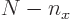forno to ex­ceed. For each such ac­cept­able pair of val­uesand, there is ex­actly one al­lowed value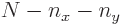. So there is ex­actly one state for each ac­cept­able pair of val­uesand. Which means that the num­ber of states is, us­ing sum­ma­tion sym­bols,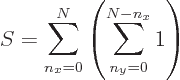The sum within the paren­the­ses is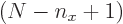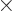1, and then the re­main­ing sum is an arith­metic se­ries [1, 21.1], pro­duc­ing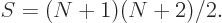Sub­sti­tut­ing in0, you get1, a sin­gle state, for1,3, three states, for2 six states and for3 ten states. So the spec­trum 4.1 shows all states.